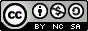# 【代码札记】The Day I Learned Haskell 4

## December 9, 2018 • 瞎折腾

### 规则

• 除了代码，你的解答必须包含一些解释性的注释。没有注释将会取得0分。博主注：我将在下面利用说明性的文本代替注释。你的注释应当完全展示出你对你的答案的理解。换句话说，一切都很公平，但是你必须证明你理解你写的代码。如果有疑问，那么就在注释中包含更多细节。
• 注释将不会计入你解答的总长度
• 类型标识不计入解答的总长度
• 空白不会计入解答的总长度。所以你没必要将你的代码全部挤在一行里。适时地使用空格，但同时保持你的代码尽可能简短。
• 我们鼓励你包含上述要求以外的函数。即：我们鼓励你将你的解答分成若干函数，如果你想这样做的话（确实，有时这样做能够达成更短的解答）。当然，额外的函数同样计入你解答的总长度（他们的类型标识除外）。
• 你最终应当提交一个名为Golf.hs的文件，其中定义的模块是Golf。所以文件首行应该是：

module Golf where
• 前三个最短的答案将赋予额外的2分，因此你最多可以获得4分。
• 其他情况下长度不是那么重要，长但是正确的答案将获得对应的解答正确的分数。额外的分数取决于他们代码的风格。

### 提示

• 尽可能多地使用标准库函数。这是这次作业的目的所在。例如使用map要比你自己造轮子方便得多。
• 特别地，尝试使用标准库函数封装递归模式，而非你自己实现递归函数。
• 或许你一开始不需要考虑长度问题，先让代码能够工作。之后再慢慢缩减长度。
• 如果有其他不明白的事情，欢迎到Piazza上弄明白。

### 任务

#### 练习 1 跳房子

skips :: [a] -> [[a]]

skips "ABCD" == ["ABCD", "BD", "C", "D"]
skips "hello!" == ["hello!", "el!", "l!", "l", "o", "!"]
skips  == []
skips [True,False] == [[True,False], [False]]
skips [] == []

##### 我的解答
skips :: [a] -> [[a]]
skips xs = if length xs == 0 then [] else map (\n -> map snd $filter (\(x,y) -> x mod n == 0)$ zip [1..length xs] xs) [1..length xs]

skips :: [a] -> [[a]]
skips [] = []
skips xs = map (\n -> map snd $filter (\(x,y) -> x mod n == 0)$ zip [1..length xs] xs) [1..length xs]

#### 练习 2 极大值

localMaxima :: [Integer] -> [Integer]

localMaxima [2,9,5,6,1] == [9,6]
localMaxima [2,3,4,1,5] == 
localMaxima [1,2,3,4,5] == []
##### 我的解答
localMaxima :: [Integer] -> [Integer]
localMaxima xs = if length xs < 3 then [] else case xs of (x:y:z:xs) -> if x < y && y > z then y : localMaxima (y:z:xs) else localMaxima (y:z:xs) 

localMaxima :: [Integer] -> [Integer]
localMAxima [] = []
localMAxima [a] = []
localMAxima [a,b] = []
localMaxima (x:y:z:xs) = if x < y && y > z then y : localMaxima (y:z:xs) else localMaxima (y:z:xs) 

#### 练习 3 直方图

histogram :: [Integer] -> String

histogram [1,1,1,5] ==
*
*
* *
==========
0123456789
histogram [1,4,5,4,6,6,3,4,2,4,9] ==
*
*
* *
****** *
==========
0123456789

"   * *    \n==========\n0123456789\n"

##### 我的解答
histogram :: [Integer] -> String
histogram xs = (helper $map (\n -> fromIntegral$ length $filter (==n) xs) [0..9]) ++ "==========\n0123456789\n" where helper ys = if foldl (+) 0 ys == 0 then [] else unlines$ (lines $helper$ map (\x -> if x > 0 then (x-1) else 0) ys) ++ [line ys]
line num = if length num == 0 then [] else case num of (z:zs) -> if z > 0 then "*" ++ line zs else " " ++ line zs

helper函数接收到列表之后，首先判断这个列表是否所有数字都是0（这里利用foldl来实现，但理论上折叠方向无所谓，但是左折叠是惰性求值的，如果列表过长可能会占用过多的内存，但是考虑到这里就只是10个数字，惰性求值也无所谓的），如果是0则说明这个直方图处理完了，返回空列表。否则哪个数字不为0，本行对应的地方就应该有个星号（由函数line完成）。随后将包含个数的列表的非0项减一，表明已经处理了一行（由匿名函数\x -> if x > 0 then (x-1) else 0完成，判断给定x是否大于0，大于就减一，否则就返回0。再由map映射到列表的每一项，实现目的），最后将所有行组成一个列表，利用unlines函数将列表拼成一个字符串，列表的每一项由换行符\n分隔。

line函数接收包含个数的列表，空列表返回空列表，否则进行模式匹配取第一个元素，如果大于0就写个星号，否则写一个空格，再将余下的列表递归调用自己。效果是遍历包含个数的列表，如果对应项为0，就是空格，若不为0，就是星号。由此可产生一个从上到下从有星号到没星号的直方图。由于我们要的是星号在下面，因此组合每一行时要把顺序调整过来。即在helper函数中最后放本行的（在最下面），先处理个数减一的。由此递归产生的效果是由下至上从有星号到无星号。

### 我的结语

1. 代码高尔夫是指利用最简短的代码实现算法。
2. 这里以中置函数调用普通函数的符号被markdown判定为行中代码，因此这里还原为了正常的函数调用形式。下同。【代码札记】The Day I Learned Haskell 4天空 Blond 采用 知识共享 署名 - 非商业性使用 - 相同方式共享 4.0 国际 许可协议进行许可。